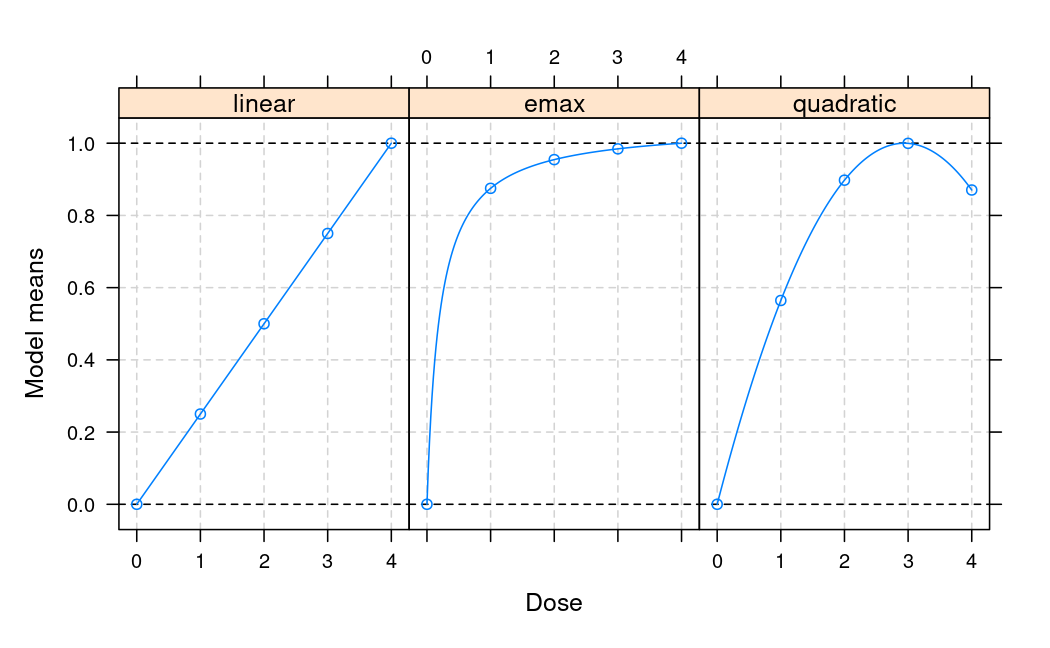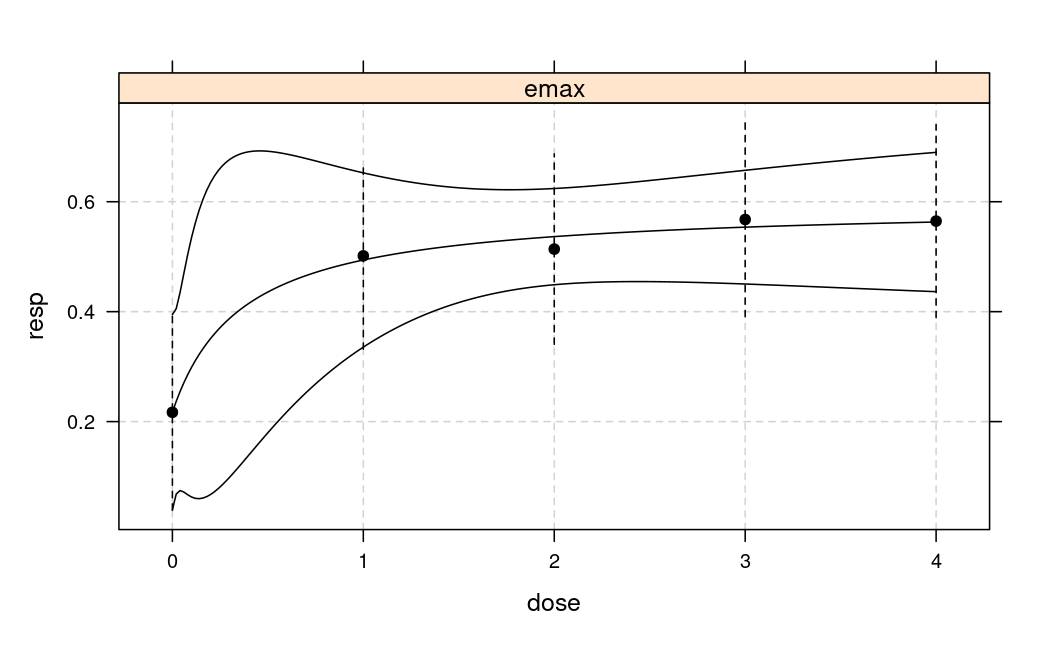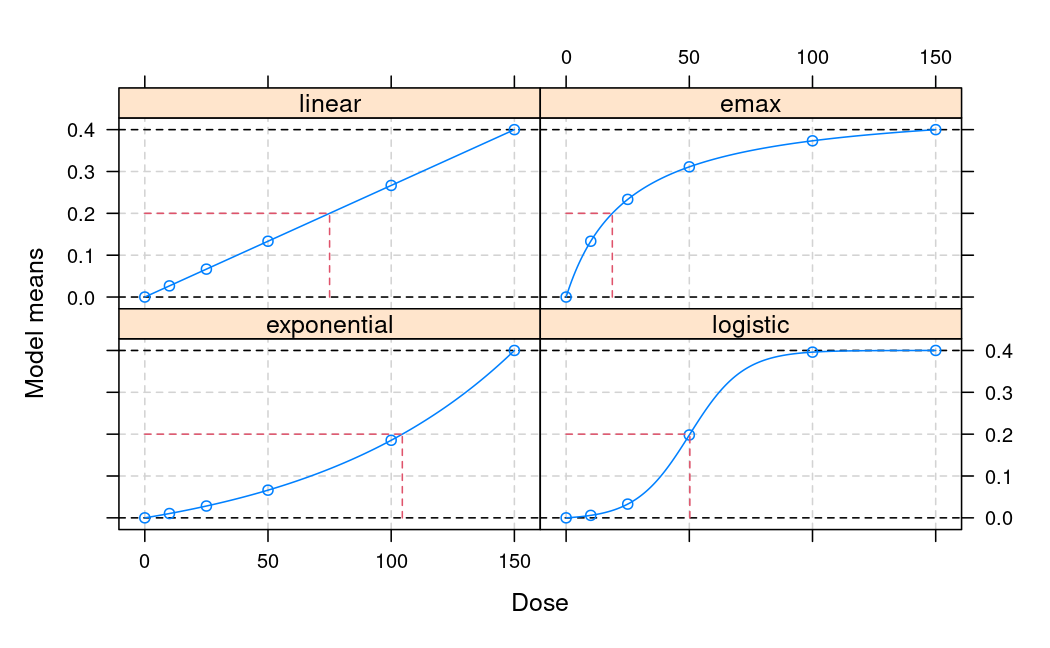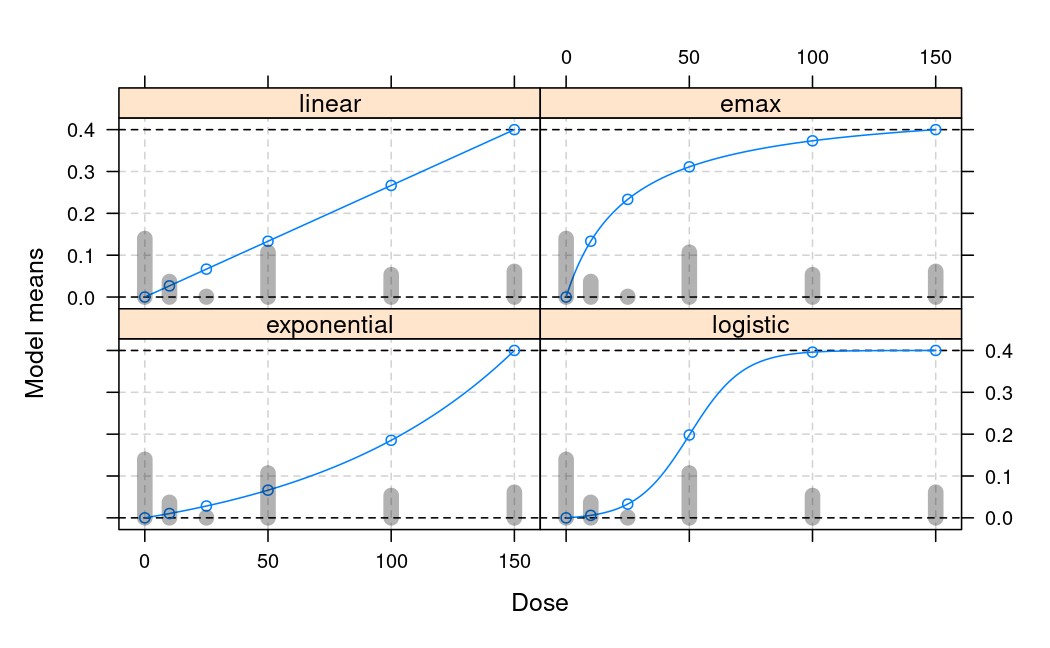# Overview DoseFinding package

The DoseFinding package provides functions for the design and analysis of dose-finding experiments (for example pharmaceutical Phase II clinical trials). It provides functions for: multiple contrast tests (MCTtest for analysis and powMCT, sampSizeMCT for sample size calculation), fitting non-linear dose-response models (fitMod for ML estimation and bFitMod for Bayesian and bootstrap/bagging ML estimation), calculating optimal designs (optDesign or calcCrit for evaluation of given designs), both for normal and general response variable. In addition the package can be used to implement the MCP-Mod procedure, a combination of testing and dose-response modelling (MCPMod) (Bretz et al. (2005), Pinheiro et al. (2014)). A number of vignettes cover practical aspects on how MCP-Mod can be implemented using the DoseFinding package. For example a FAQ document for MCP-Mod, analysis approaches for normal and binary data, sample size and power calculations as well as handling data from more than one dosing regimen in certain scenarios.

Below a short overview of the main functions.

## Perform multiple contrast test

library(DoseFinding)
data(IBScovars)
head(IBScovars)
  gender      resp dose
1      1 1.5769231    1
2      1 0.6833333    3
3      1 0.2857143    0
4      1 0.6307692    3
5      1 0.1428571    2
6      1 0.1571429    1
## perform (model based) multiple contrast test
## define candidate dose-response shapes
models <- Mods(linear = NULL, emax = 0.2, quadratic = -0.17,
doses = c(0, 1, 2, 3, 4))
## plot models
plot(models)## perform multiple contrast test
## functions powMCT and sampSizeMCT provide tools for sample size
## calculation for multiple contrast tests
test <- MCTtest(dose, resp, IBScovars, models=models,
test
Multiple Contrast Test

Contrasts:
0 -0.616 -0.889    -0.815
1 -0.338  0.135    -0.140
2  0.002  0.226     0.294
3  0.315  0.252     0.407
4  0.638  0.276     0.254

Contrast Correlation:
linear     1.000 0.768     0.843
emax       0.768 1.000     0.948

Multiple Contrast Test:
emax       3.208 0.00160
linear     2.640 0.00844

## Fit non-linear dose-response models here illustrated with Emax model

fitemax <- fitMod(dose, resp, data=IBScovars, model="emax",
bnds = c(0.01,5))
## display fitted dose-effect curve
plot(fitemax, CI=TRUE, plotData="meansCI")## Calculate optimal designs, here illustrated for target dose (TD) estimation

## optimal design for estimation of the smallest dose that gives an
## improvement of 0.2 over placebo, a model-averaged design criterion
## is used (over the models defined in Mods)
doses <- c(0, 10, 25, 50, 100, 150)
fmodels <- Mods(linear = NULL, emax = 25, exponential = 85,
logistic = c(50, 10.8811),
doses = doses, placEff=0, maxEff=0.4)
plot(fmodels, plotTD = TRUE, Delta = 0.2)weights <- rep(1/4, 4)
desTD <- optDesign(fmodels, weights, Delta=0.2, designCrit="TD")
desTD
Calculated TD - optimal design:
0      10      25      50     100     150
0.34960 0.09252 0.00366 0.26760 0.13342 0.15319 
plot(desTD, fmodels)